## IntroductionMicrosoft Solver Foundation is an exciting new .NET based optimization platform that includes solvers for Linear Programming (LP), Mixed Integer Programming (MIP), Quadratic Programming (QP) and CSP (Constraint Programming) problems. Included in version 1.1 is the high-performance state-of-the-art multi-threaded Gurobi MIP solver and plugin capabilities for third party solvers such as Cplex and Mosek. We can provide services to help you design, prototype, develop and implement high-performance, scalable and reliable optimization models on this framework. Models developed in Microsoft Solver Foundation can be integrated seamlessly in your .NET applications and services.

### Excel plug-in

MSF includes a simple modeling language OML. This language can be used from the MSF API but also from the Excel plug-in. Here we make available a document describing experiences with and hints for using the Excel/OML combination. We do this using a number of small models. Note that MSF also contains a wealth of API's to be called from user programs.

Below is a list of toy models that are discussed in the above document. The size of the models is kept small so they can be solved with the Standard Edition of Microsoft Solver Foundation. These small toy examples are used to demonstrate certain features of OML. Real world applications are often too large, too specific and too messy to be very useful for demonstrations, while these smaller examples show features that can be used directly in larger contexts.

#### Simple transportation modelModel number one of the GAMS model ibrary is this transportation model from the famous book by George Dantzig (Linear Programming and Extensions, Princeton University Press, 1963). It has been slightly changed to introduce dual degeneracy, so several optimal solutions exist. Although this linear programming problem is very small it demonstrates indexing and scalable formulations. We argue that OML is a step forward from using Excel Solver as it shows rather than hides the structure of the model.

#### Sparse data modelsGAMS and Excel work with sparse data: missing data or empty cells are considered to be zero when using operations such as summations. The Data Binding facility in the MSF Excel plug-in requires a "dense" data representation. Here we show two possible solutions: first in the diet model we augment the data with the zero values. In the maximum flow network model we show how we can enumerate the set of arcs so we don't need to specify non-existing arcs.

#### CSP Model: Magic SquaresConstructing Magic Squares is difficult to model efficiently using Mixed Integer Programming. A magic square has numbers 1,..,n2 placed on the board such that the rows, the columns and the diagonals add up to the same value. The problem can be expressed much more natural with a CSP solver using the all-different constraint. Some non-standard solver options were required to achieve better performance.

#### CSP Model: The Social Golfer Problem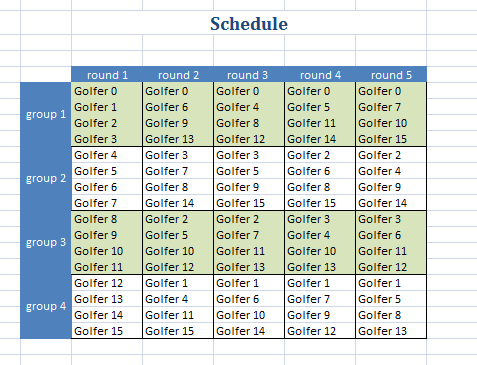Constructing a schedule for a golf trip where players don't play each other more than once is a difficult problem. Here are some formulations for N=16 golfers playing 5 rounds with 4 foursomes. These models solve fairly easily. The same problem with N=32 golfers, 10 rounds is very difficult and I was not able to solve that instance.

The models are implemented as CSP models: they are more compact than the corresponding MIP models. Scheduling models are a well-know application area for CSP algorithms.

#### Traveling Salesman Problem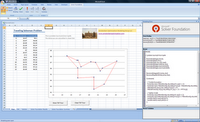The traveling salesman problem (TSP) is a famous difficult problem. A salesman has to visit a number of cities and return to the starting point. The goal is to minimize the total distance traveled. The TSP problem is probably the most studied problem in the OR field. Here we solve a small instance as a MIP.

The current state-of-the-art MIP solvers can solve TSPs up to about 50 cities, and find good solutions for even larger problems. Of course specialized algorithms and solvers are capable of handling larger instances.

#### CSP Model: Langford's Problem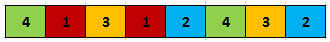Computing Langford sequences can be specified easily using the all-different constraint. The problem is to place the numbers (1,1,2,2,3,3,4,4) such that equal numbers k have k other numbers in between, e.g. (4,1,3,1,2,4,3,2).

#### CSP Model: Tiling SquaresTiling squares with an upper limit on how many duplicate tiles can be used. The (7x7) problem below is easy we assume we know that we can cover the (7x7) are with tiles (4x4), (3x3), (3x3), (2x2), (2x2), (2x2), (1x1), (1x1), (1x1). The (9x9) problem is more difficult: we have an inventory of three tiles of each size (1x1) through (8x8) but not every tile has to be used. The big (19x19) problem is solved with a pool of just two tiles of each size (1x1) through (16x16). Modeling tricks to reduce symmetry are applied to be able to solve the larger instances.

#### QP: Efficient Porfolio Frontier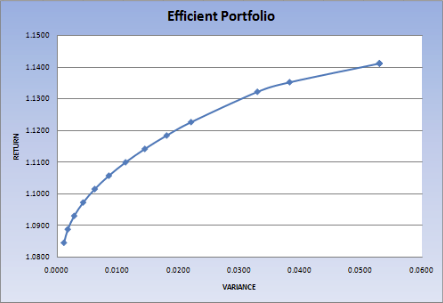Quadratic Programming (QP) models can be used to model a portfolio optimization problem. When we want to draw an efficient frontier a simple algorithm is to solve several QP's. Unfortunately there are no looping constructs in OML: we can form and solve only a single model with the Excel plug-in. In this example we combine the QP's into a single large QP.

#### QP vs LP: Optimal Porfolio SelectionIn this model we compare an LP formulation with a QP formulation for the portfolio model. The multiple models are handled by a piece of C# code which is compiled into a DLL. Inside the C# code we express the models in OML. The DLL is called from Excel via some VBA code. The output reports in the form of graphs are created in Excel.

#### Column Generation: Cutting Stock example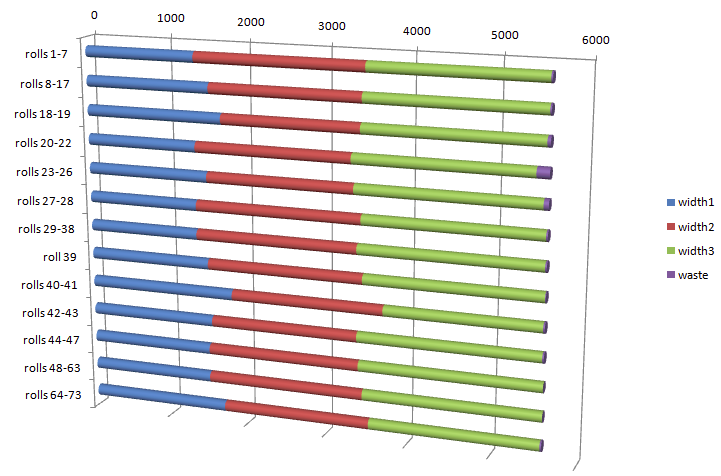A remarkable algorithm to solve Cutting Stock problems is based on Column Generation, where new variables (columns) are added to an LP model. In the document above a simple implementation of a column generation approach is implemented using MSF. The method is applied to a standard cutting stock problem where rolls of raw material have to be cut while minimizing waste.

#### Job Shop SchedulingJob Shop Scheduling problems can be difficult to solve. An example is ft10 with 10 jobs and 10 machines. An optimal solution for this problem was not known for 25 years. Nowadays with the best MIP solvers such as Gurobi and Cplex we can solve this problem formulated as a standard MIP model in less than 5 minutes. Some VBA code has been developed to produce GANTT charts in Excel.